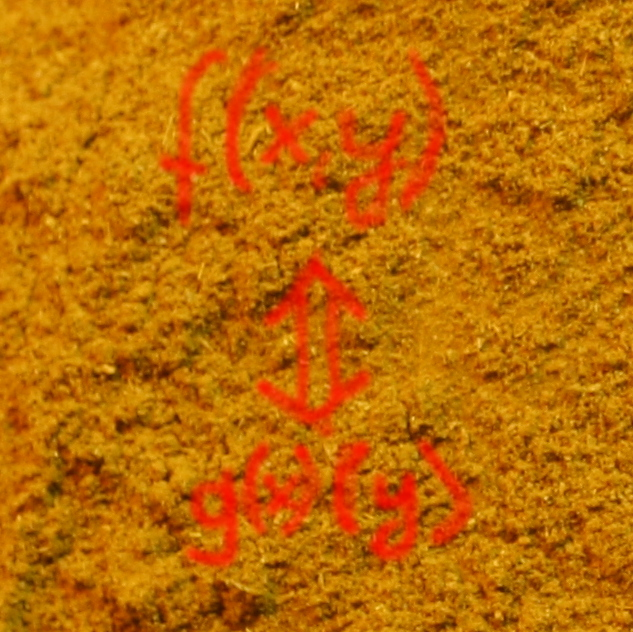## Currying

### General IdeaIn mathematics and computer science, currying is the technique of breaking down the evaluation of a function that takes multiple arguments into evaluating a sequence of single-argument functions. Currying is also used in theoretical computer science, because it is often easier to transform multiple argument models into single argument models.

### Composition of Functions

We define the composition h of two functions f and g

$h(x) = g(f(x))$

in the following Python example.

The composition of two functions is a chaining process in which the output of the inner function becomes the input of the outer function.

def compose(g, f):
def h(x):
return g(f(x))
return h


We will use our compose function in the next example. Let's assume, we have a thermometer, which is not working accurately. The correct temperature can be calculated by applying the function readjust to the temperature values. Let us further assume that we have to convert our temperature values from Celsius to Fahrenheit. We can do this by applying compose to both functions:

def celsius2fahrenheit(t):
return 1.8 * t + 32

def readjust(t):
return 0.9 * t - 0.5

convert = compose(readjust, celsius2fahrenheit)

print(convert(10), celsius2fahrenheit(10))

44.5 50.0


The composition of two functions is generally not commutative, i.e. compose(celsius2fahrenheit, readjust) is different from compose(readjust, celsius2fahrenheit)

convert2 = compose(celsius2fahrenheit, readjust)

print(convert2(10), celsius2fahrenheit(10))

47.3 50.0


convert2 is not a solution to our problem, because it is not readjusting the original temperatures of our thermometer but the transformed Fahrenheit values!

### Example Currency Conversion

In our chapter on Magic Functions we had an exercise on currency conversion.

### "compose" with Arbitrary Arguments

The function compose which we have just defined can only copy with single-argument functions. We can generalize our function compose so that it can cope with all possible functions, along with an example using a function with two parameters.

def compose(g, f):
def h(*args, **kwargs):
return g(f(*args, **kwargs))
return h

def BMI(weight, height):
return weight / height**2

def evaluate_BMI(bmi):
if bmi < 15:
return "Very severely underweight"
elif bmi < 16:
return "Severely underweight"
elif bmi < 18.5:
return "Underweight"
elif bmi < 25:
return "Normal (healthy weight)"
elif bmi < 30:
return "Overweight"
elif bmi < 35:
return "Obese Class I (Moderately obese)"
elif bmi < 40:
return "Obese Class II (Severely obese)"
else:
return "Obese Class III (Very severely obese)"

f = compose(evaluate_BMI, BMI)

again = "y"
while again == "y":
weight = float(input("weight (kg) "))
height = float(input("height (m) "))
print(f(weight, height))
again = input("Another run? (y/n)")

Normal (healthy weight)


### Currying Function with an Arbitrary Number of Arguments

One interesting question remains: How to curry a function across an arbitrary and unknown number of parameters?

We can use a nested function to make it possible to "curry" (accumulate) the arguments. We will need a way to tell the function calculate and return the value. If the funtions is called with arguments, these will be curried, as we have said. What if we call the function without any arguments? Right, this is a fantastic way to tell the function that we finally want to the the result. We can also clean the lists with the accumulated values:

def arimean(*args):
return sum(args) / len(args)

def curry(func):
# to keep the name of the curried function:
curry.__curried_func_name__ = func.__name__
f_args, f_kwargs = [], {}
def f(*args, **kwargs):
nonlocal f_args, f_kwargs
if args or kwargs:
f_args += args
f_kwargs.update(kwargs)
return f
else:
result = func(*f_args, *f_kwargs)
f_args, f_kwargs = [], {}
return result
return f

curried_arimean = curry(arimean)
curried_arimean(2)(5)(9)(4, 5)
# it will keep on currying:
curried_arimean(5, 9)
print(curried_arimean())

# calculating the arithmetic mean of 3, 4, and 7
print(curried_arimean(3)(4)(7)())

# calculating the arithmetic mean of 4, 3, and 7
print(curried_arimean(4)(3, 7)())

5.571428571428571
4.666666666666667
4.666666666666667


Let's compare it with the result of the original arimean function:

print(arimean(2, 5, 9, 4, 5, 5, 9))
print(arimean(3, 4, 7))
print(arimean(4, 3, 7))

5.571428571428571
4.666666666666667
4.666666666666667


Including some prints might help to understand what's going on:

def arimean(*args):
return sum(args) / len(args)

def curry(func):
# to keep the name of the curried function:
curry.__curried_func_name__ = func.__name__
f_args, f_kwargs = [], {}
def f(*args, **kwargs):
nonlocal f_args, f_kwargs
if args or kwargs:
print("Calling curried function with:")
print("args: ", args, "kwargs: ", kwargs)
f_args += args
f_kwargs.update(kwargs)
print("Currying the values:")
print("f_args: ", f_args)
print("f_kwargs:", f_kwargs)
return f
else:
print("Calling " + curry.__curried_func_name__ + " with:")
print(f_args, f_kwargs)

result = func(*f_args, *f_kwargs)
f_args, f_kwargs = [], {}
return result
return f

curried_arimean = curry(arimean)
curried_arimean(2)(5)(9)(4, 5)
# it will keep on currying:
curried_arimean(5, 9)
print(curried_arimean())

Calling curried function with:
args:  (2,) kwargs:  {}
Currying the values:
f_args:  
f_kwargs: {}
Calling curried function with:
args:  (5,) kwargs:  {}
Currying the values:
f_args:  [2, 5]
f_kwargs: {}
Calling curried function with:
args:  (9,) kwargs:  {}
Currying the values:
f_args:  [2, 5, 9]
f_kwargs: {}
Calling curried function with:
args:  (4, 5) kwargs:  {}
Currying the values:
f_args:  [2, 5, 9, 4, 5]
f_kwargs: {}
Calling curried function with:
args:  (5, 9) kwargs:  {}
Currying the values:
f_args:  [2, 5, 9, 4, 5, 5, 9]
f_kwargs: {}
Calling arimean with:
[2, 5, 9, 4, 5, 5, 9] {}
5.571428571428571